What is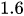as a fraction?Rodrigo

Solution:

Step:1 To convert a decimal into fraction, following procedure is used.

1. Observe, after how many places from the right a decimal is placed.

2. Divide the number after removing the decimal from it by a number as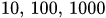etc number of zeros after 1 depends upon the place from the right a decimal is placed.

3. Convert the fraction obtained in step 2 in simplest form.. If the denominator is greater than the numerator then the fraction is proper and if the numerator is greater than denominator then the fraction is improper.

Step:2 Similarly, to convert a fraction into a decimal, following procedure is used.

1. Observe the denominator of the fraction, if the denominator is a number which can be written in the form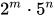, where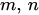are non-negative integers, then the fraction can be converted in a terminating decimal otherwise it is a non-terminating decimal.

2. Divide the numerator by denominator.

3. If the division is non-terminating or the division is too long, then the decimal expansion can be rounded up to 2 to 3 decimal places, which gives the approximate value of fraction.

Step:3 Conversion of 1.6 as a fraction:

1.In the decimal number 1.6, decimal is placed after one number from the right.

2. Divide the number 16 by 10.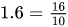3. Convert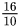in simplest form.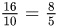Answer: 1.6 is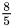as a fraction.

Similar Problems:

Problem 1: Convert 1.7 as a fraction.

Solution:

Step:1 1.7 has a decimal after one digit from right.

Divide the number removing decimal from it, by 10.

Step:2 Conversion of 1.7 into a fraction is as follows: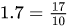Answer: 1.7 is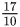as a fraction.

Problem 2: Convert 1.2 as a fraction.

Solution:

Step:1 1.2 has a decimal after one digit from the right.

Divide the number removing decimal from it, by 10.

Step:2 Conversion of 1.2 into a fraction is as follows: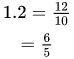Answer: 1.2 is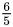as a fraction.

<math xmlns='http://www.w3.org/1998/Math/MathML'>
<mrow>
<mfrac>
<mn>8</mn>
<mn>5</mn>
</mfrac>
</mrow>
[/itex]

Need homework help now?

tutors online

Related Questions
(t) in °C of the pot of soup at time t (in The initial temperature of a pot of soup is 80°C. The temperature minutes) satisfies the differential equation dT di T-20 10 (a) Solve the differential equation, and find an explicit expression for the function (). By separating variables, integrating and manipulating arbitrary constants, . We are also told that so that and so . ...
Notes
Me

OR

Don't have an account?

Join OneClass

Access over 10 million pages of study
documents for 1.3 million courses.

Join to view

OR

By registering, I agree to the Terms and Privacy Policies
Just a few more details

So we can recommend you notes for your school.